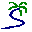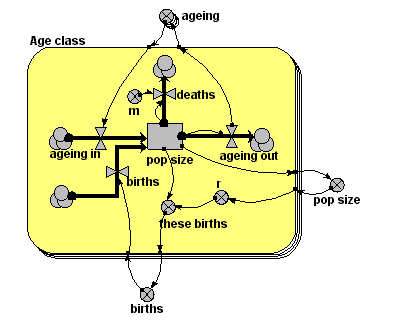# Model entry

Age-class model of population dynamics, using multiple-instance submodel - Model catalogue - Simulistics.com

 Search Simulistics Model catalogue Listed by keyword Listed by ID Listed by title Listed by date added

# Age-class model of population dynamics, using multiple-instance submodel

Model : ageclass5
Simile version : 3.1+
Keywords : Age-class modelling ; Population dynamics ;

## Description

This models a population of animals in terms of four age-classes. For each age-class, we use a compartment (state variable) to represent the number of individuals in that class.

This version uses a four-instance submodel to represent the four age-classes. The ageing flow (from one class to the next) thus has to be handled by transferring the value for the outflow from each class to be assigned to the inflow for the next class. In this model, this is done by placing all four outflow values in an array outside the submodel, then selecting the appropriate value from this array for each inflow.

## Files

### Model file

Click on the icon to download the model file. (You will need Simile to examine and run the model. A free evaluation version is available from the products page.)

Some browsers may attempt to display the model file, rather than open it in Simile; in this case, use the browser back button to return to this page, and use the context menu (invoked by right-clicking on the link) to save the target file to disk.ageclass5.sml

## Diagram## Equations

```Equations in Desktop
births      = sum([these_births])
pop size    = sum([pop_size])
ageing      = [ageing_out]

Equations in Age class
pop size:     initial value = element([2,0,0,0],index(1))
births      = if index(1) == 1 then births else 0
ageing out  = element([1,0.2,0.1,0],index(1))*pop_size
deaths      = m*pop_size
ageing in   = if index(1)>1 then element([ageing],index(1)-1)else 0
m           = element([0.05,0.01,0.01,0.05],index(1))
r           = element([0,0.05,0.2,0.1],index(1))*(1-pop_size/100)
these births= r*pop_size
```

## Results

||